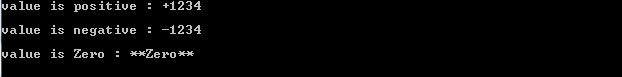Pranay Rana: Format Number To Display

## Wednesday, June 6, 2012

### Format Number To Display

Number of time the end user / client require to display numeric data in different format. In this post I am going to discuss about the various type of the custom format that provided by C#.net to achieve requirement.
Here I am going to discuss each format one by one

"0" Custom Specifier
ToString("00000") - format put the leading 0 when number get display if digits in number less than the number of zero specified. when the below code is get executed output display 01234 because the number of digit less than the number of zero.
```double value;
value = 1234;
Console.WriteLine("Format 1:  " + value.ToString("00000"));
Console.WriteLine();
```
ToString("00.00") - format do same thing as above replace zero if the number digit less , but the zero after decimal point allows to display digit equals number of zero after decimal if the number of digit less than display zero in place of that. output of the following code is 01.24 i.e only 2 digit allowed after decimal point. Note : - decimal point is display as per specified culture.
```value = 1.235;
Console.WriteLine("Format 2:  " + value.ToString("00.00",
CultureInfo.InvariantCulture));
Console.WriteLine();
```
ToString("0,0") - format cause to display comma between number. here when the following code is get executed , is get display after every three digit 1,234,567,890. Note : - in this comma get replace by the culture.
```value = 1234567890;
Console.WriteLine("Format 3:  " + value.ToString("0,0",
CultureInfo.InvariantCulture));
Console.WriteLine();
```
ToString("0,0.0") - format is combination of above format.
```value = 1234567890.123456;
Console.WriteLine("Format 4:  " + value.ToString("0,0.0",
CultureInfo.InvariantCulture));
Console.WriteLine();
```

Output

"#" Custom Specifier
This specifier does same thing as "0" specifier do but the basic difference is it doesn't anything if the number is no equal to # where as "0" specifier replace 0 if digit not exists.
ToString("#####") or ToString("#") - format allow to replace each digit by #, output of below code execution is 9867752985 which is differ from "0" specifier.
```value = 9867752985;
Console.WriteLine(value.ToString("#####"));
Console.WriteLine(value.ToString("#"));
Console.WriteLine();
```
Below code does same as above but format number by replace each # by each digit. so the output of the code is 98-67-75.
```value = 986775;
Console.WriteLine(value.ToString("[##-##-##]"));
Console.WriteLine();
```
Below code execution again format number and output display it as (986) 77-52985
```value = 9867752985;
Console.WriteLine(value.ToString("(###) ###-####"));
Console.WriteLine();
```
Output"." Custom Specifier
This specifier is already discuss with the "0" specifier this useful to display numeric number if the digit is not present than 0 get display on that place i.e at the start or end. so the output of below code is "01.20" and if I set value = 12 than the output is "12.00". But here I set culture info so that output is "01,20" so "." get replace by ",".
```value=1.2
Console.WriteLine(value.ToString("00.00",CultureInfo.CreateSpecificCulture("da-DK")));
```

"%" Custom Specifier
Format cause the number to display with "%" and multiply number with 100. so if the number is 0.86 after applying % specifier it get display as "8.6%" if the number is 86 than output will be "8600%". Below code execution does the same thing
```value = .086;
Console.WriteLine(value.ToString("#0.##%", CultureInfo.InvariantCulture));
Console.WriteLine();

value = .869;
Console.WriteLine(value.ToString("00.##%", CultureInfo.InvariantCulture));
Console.WriteLine();

value = 86;
Console.WriteLine(value.ToString("#0.##%", CultureInfo.InvariantCulture));
Console.WriteLine();
```
Oputput"‰" Custom Specifier
per mille character (‰ or \u2030) format multiply the number by 1000. So the output of below code execution is 3.54‰ . But I don't think this format is useful somewhere.
```value = .00354;
string perMilleFmt = "#0.## " + '\u2030';
Console.WriteLine(value.ToString(perMilleFmt, CultureInfo.InvariantCulture));
```

"E" and "e" Custom Specifiers
This cause number to be display in scientific notation format with the "E or e" symbol.
```value = 86000;
Console.WriteLine(value.ToString("0.###E+0", CultureInfo.InvariantCulture));
Console.WriteLine();

Console.WriteLine(value.ToString("0.###E+000", CultureInfo.InvariantCulture));
Console.WriteLine();

value = -80;
Console.WriteLine(value.ToString("0.###E-000", CultureInfo.InvariantCulture));
Console.WriteLine();
```
Output";" Section Separator
This allow to display number according to number sign. As you can see in below code the fmt variable which is format I am going to apply on my number here first format before ; is for positive number , second format is for negative number and last format is for the zero value.
Basically its "Positive;negative;zero" format.
You can see the what it does in output of this code.
```double posValue = 1234;
double negValue = -1234;
double zeroValue = 0;

string fmt = "+##;-##;**Zero**";

Console.WriteLine("value is positive : " + posValue.ToString(fmt));
Console.WriteLine();

Console.WriteLine("value is negative : " +negValue.ToString(fmt));
Console.WriteLine();

Console.WriteLine("value is Zero : " + zeroValue.ToString(fmt));
Console.WriteLine();
```
OutputSource :  http://msdn.microsoft.com/en-us/library/0c899ak8.aspx